# Number Properties - Definition with Examples

The Complete K-5 Math Learning Program Built for Your Child

• 40 Million Kids

Loved by kids and parent worldwide

• 50,000 Schools

Trusted by teachers across schools

• Comprehensive Curriculum

Aligned to Common Core

## Number Properties

The four main number properties are:

## Commutative property:

The commutative property states that the numbers on which we perform the operation can be moved or swapped from their position without making any difference to the answer.

This property holds true for addition and multiplication, but not for subtraction and division.

Example of the commutative property of addition

3 + 5 = 5 + 3 = 8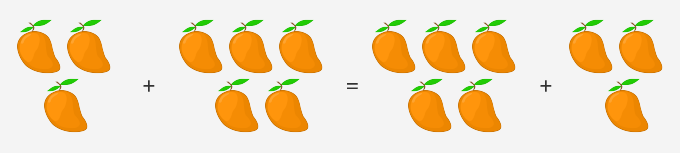Hence, the commutative property of addition for any two real numbers a and b is:

a + b = b + a

Example of the commutative property of multiplicationHence, the commutative property of multiplication for any two real numbers a and b is:

a x b = b x a

We can also say that in commutative property, the numbers can be added or multiplied to each other in any order without changing the answer.

## Associative Property:

The associative property gets its name from the word “Associate” and it refers to the grouping of numbers. This property states that when three or more numbers are added (or multiplied), the sum(or product) is the same regardless of the grouping of the addends (or multiplicands).

For example:

(3 + 4) + 5 = (4 + 5) + 3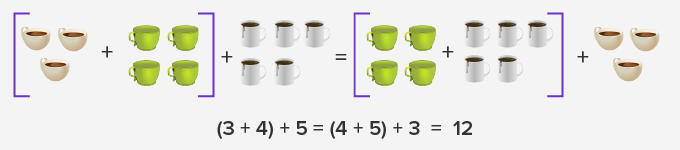( 4 x 7 ) x 5 = ( 4 x 5 ) x 7 =140

## Identity Property:

 Additive identity Multiplicative identity Additive identity is a number, which when added to any number, gives the sum as the number itself. This means, the additive identity is “0” as adding 0 to any number, gives the sum as the number itself.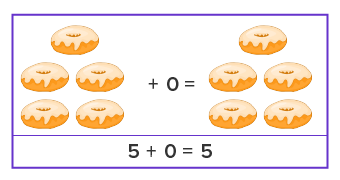Multiplicative identity is a number, which when multiplied by any number, gives the product as the number itself. This means, the multiplicative identity is “1” as multiplying any number by 1, gives the product as the number itself.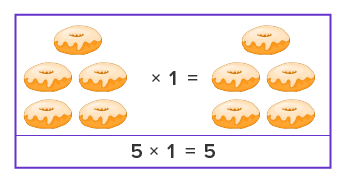## Distributive Property:

Distributive property helps us to simplify the multiplication of a number by a sum or difference. As the name suggests, it distributes the expression.

For example:  a x (b + c)

Using distributive property, we can expand the expression as:Example of distributive property using addition Example of distributive property using subtraction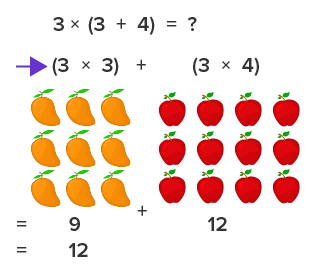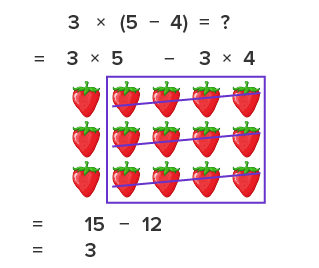Fun Facts The additive identity property is also called the zero property of addition. The commutative property gets its name from the word commutes, meaning move around.

Won Numerous Awards & Honors